Ex 7.3

Chapter 7 Class 9 Triangles
Serial order wise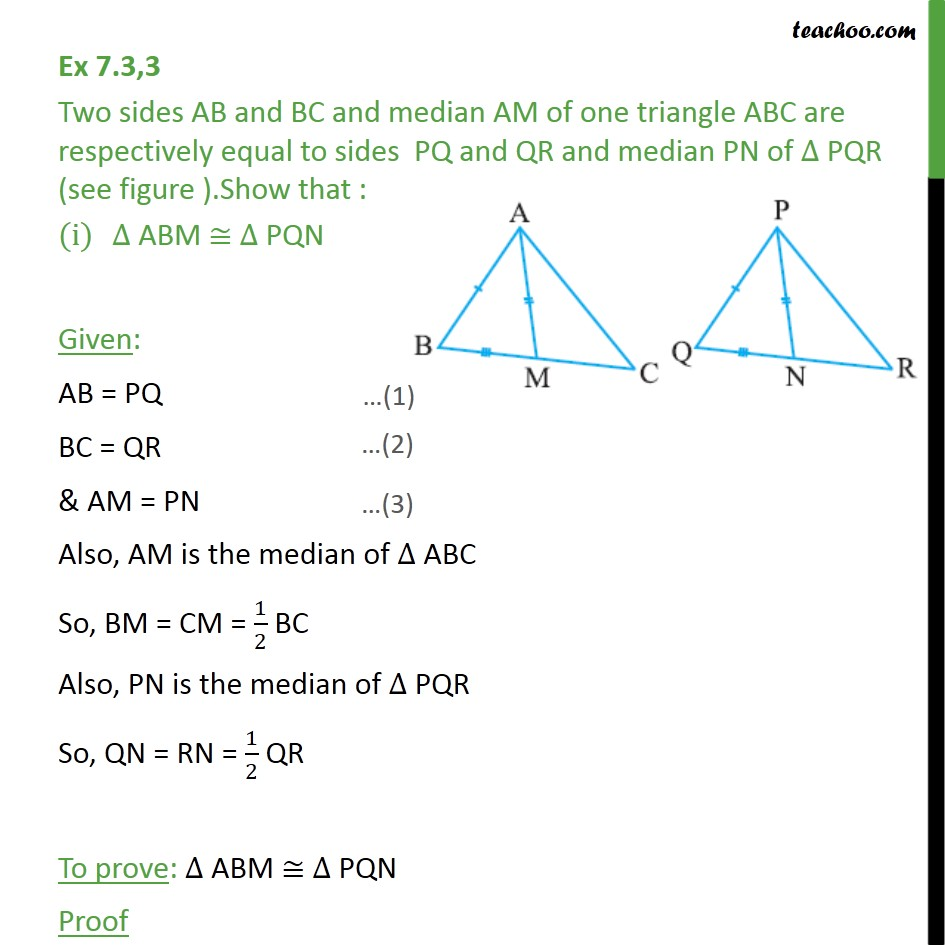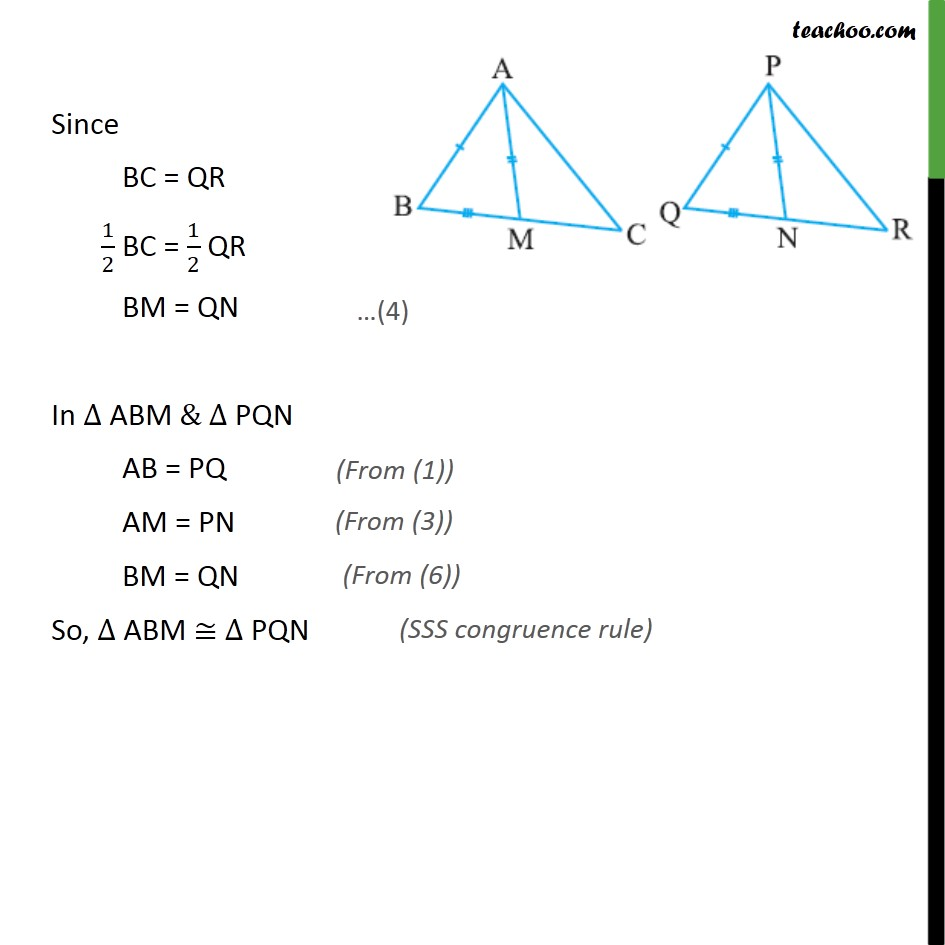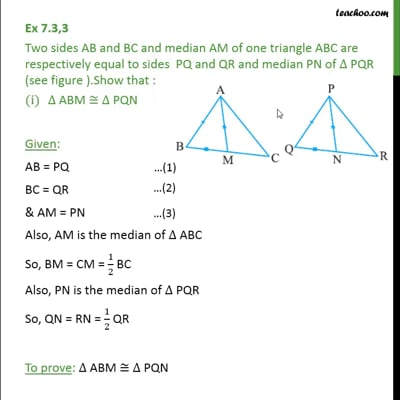This video is only available for Teachoo black users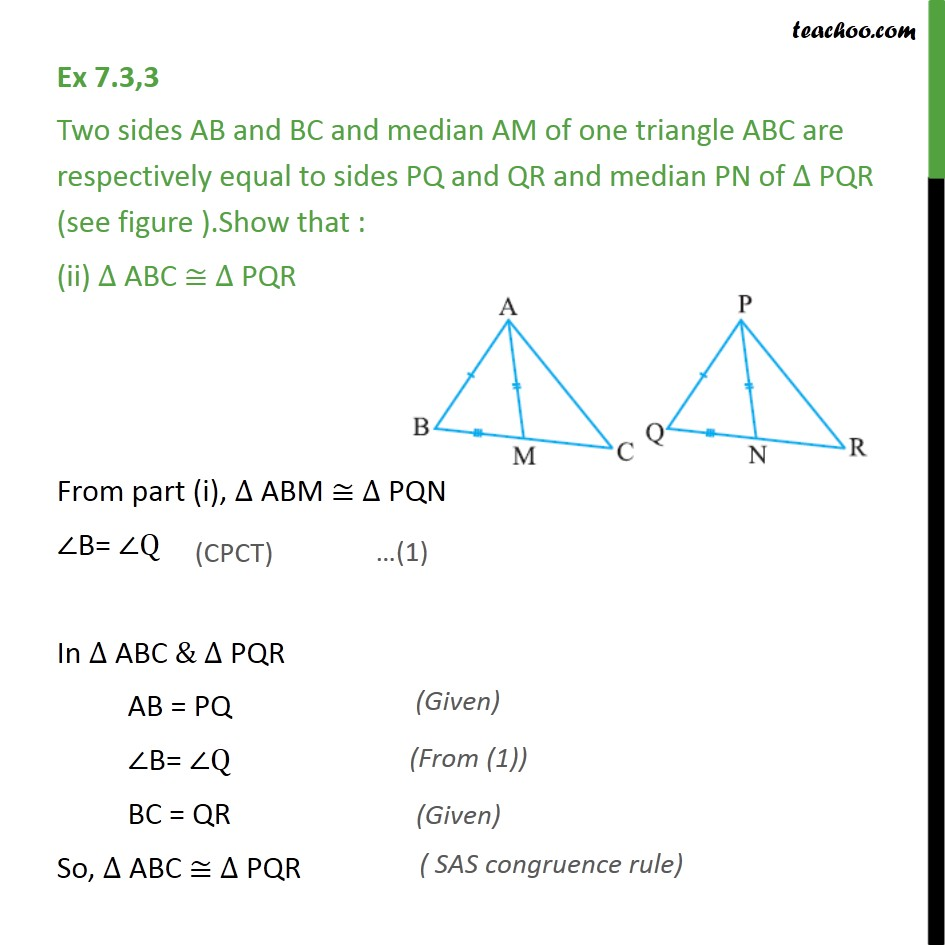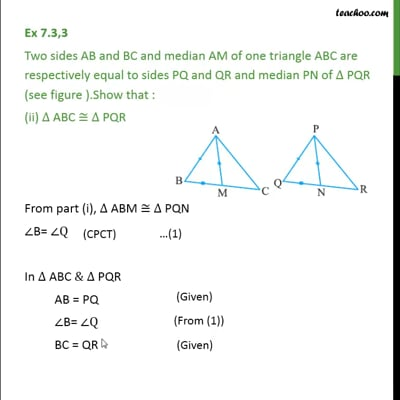This video is only available for Teachoo black users

Maths Crash Course - Live lectures + all videos + Real time Doubt solving!

### Transcript

Ex 7.3,3 Two sides AB and BC and median AM of one triangle ABC are respectively equal to sides PQ and QR and median PN of PQR (see figure ).Show that : ABM PQN Given: AB = PQ BC = QR & AM = PN Also, AM is the median of ABC So, BM = CM = 1/2 BC Also, PN is the median of PQR So, QN = RN = 1/2 QR To prove: ABM PQN Proof Since BC = QR 1/2 BC = 1/2 QR BM = QN In ABM & PQN AB = PQ AM = PN BM = QN So, ABM PQN Ex 7.3,3 Two sides AB and BC and median AM of one triangle ABC are respectively equal to sides PQ and QR and median PN of PQR (see figure ).Show that : (ii) ABC PQR From part (i), ABM PQN B= Q In ABC & PQR AB = PQ B= Q BC = QR So, ABC PQR